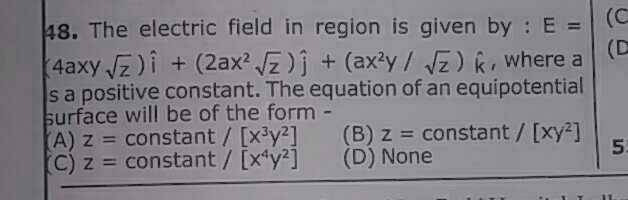# Equipotential surface and electric field

Jahnavi

## Homework Statement## The Attempt at a Solution

I know the relation between electric field and electric potential . I can also find Electric field if expression for potential is given and vica versa . But I do not know how to work with electric field and equipotential surfaces . Since this is an MCQ , I believe there must be some simple underlying concept involved in it .

Is there a way to get the expression for potential from the equipotential surface or vica versa ?

#### Attachments

Homework Helper
Gold Member
I know the relation between electric field and electric potential .
If you know the relation, then use the given field components to find an expression for the potential. Sometimes even MCQs require you to do work. You could try dimensional analysis, but you must be sure you know what you're doing because of (d) being a possibility.

Jahnavi
If you know the relation, then use the given field components to find an expression for the potential.

Sorry . I am not sure how to do this . Although if expression for potential is given then using partial differentiation electric field can be obtained .

Ex= -∂V/∂x

But electric field is given in the problem .

Homework Helper
Gold Member
But electric field is given in the problem .
I agree. You are looking for the potential, not the electric field. If $$\frac{\partial V(x,y,z)}{\partial x}=-4axy\sqrt{z}$$ what could ##V(x,y,z)## possibly be in the most general case?

•Jahnavi
Jahnavi
If $$\frac{\partial V(x,y,z)}{\partial x}=-4axy\sqrt{z}$$ what could ##V(x,y,z)## possibly be in the most general case?

-2ax2y√z

I think this will also be the expression if I do the same thing in Y and Z direction .

Are the options given in the question expression for potentials ? If yes , then option C) looks correct . Is that right ?

But the question asks to find equipotential surfaces . "Equation of a surface" and "expression for potential" are two different things . Isn't it ?

Last edited:
Homework Helper
Gold Member
You are not done yet. The potential could be ##V(x,y,z)=-2ax^2y\sqrt{z}+f(y,z)## and the x-component of the electric field would still be ##E_x=4axy\sqrt{z}##. If this is the case, then the correct answer could be (d). So you need to do more work with the other two components that are given to you.
"Equation of a surface" and "expression for potential" are two different things . Isn't it ?
Yes. If you have an expression for ##V(x,y,z)##, you can solve the equation ##V(x,y,z) = constant## for ##z## and compare your answer with the three possible answers.

•Jahnavi
Jahnavi
You are not done yet. The potential could be ##V(x,y,z)=-2ax^2y\sqrt{z}+f(y,z)## and the x-component of the electric field would still be ##E_x=4axy\sqrt{z}##. If this is the case, then the correct answer could be (d).

You are rightSo you need to do more work with the other two components that are given to you.

What should I do now ?

The same thing you did in the x-direction, but now do it in the y-direction. You know that $$\frac{\partial V(x,y,z)}{\partial y}=-2ax^2\sqrt{z}$$ and you have found that ##V(x,y,z)=-2ax^2y\sqrt{z}+f(y,z)##. Put it together and see what you can say about the y-dependence of ##f(y,z)##. Then repeat with the z-dependence.
•